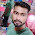### Program 131: To Transpose a given Matrix

Program 131:

```#include<stdio.h>
main()
{
int i,j,rows,col,temp;
printf("Enter number of rows and columns of a matrix\n");
scanf("%d %d",&rows,&col);

int a[rows][col],t[rows][col];
//Taking input of matrix
printf("Enter Matrix 1\n");
for(i=0;i<rows;i++)
{
for(j=0;j<col;j++)
{
scanf("%d",&a[i][j]);
}
}
printf("Given /matrix is\n");
for(i=0;i<rows;i++)
{
for(j=0;j<col;j++)
{
printf("%d\t",a[i][j]);
}

printf("\n");
}
//Exchanging rows and columns
temp=rows;
rows=col;
col=temp;
//Transpose of matrix
for(i=0;i<rows;i++)
{
for(j=0;j<col;j++)
{
t[i][j]=a[j][i];
}
}

printf("Transpose of above matrix is\n");

for(i=0;i<rows;i++)
{
for(j=0;j<col;j++)
{
printf("%d\t",t[i][j]);
}

printf("\n");
}

}

```
Explanation:

//Coming Soon

Output:

#### 1 comment:

1.factorial hundred In the last few days, the “factorial of 100” is one of the top subjects and a lot of maths geeks compute it using voice assistants such as Alexa, Shiri, etc.

factorial hundred In the last few days, the “factorial of 100” is one of the top subjects and a lot of maths geeks compute it using voice assistants such as Alexa, Shiri, etc.

factorial hundred In the last few days, the “factorial of 100” is one of the top subjects and a lot of maths geeks compute it using voice assistants such as Alexa, Shiri, etc.

 Donate Buy me a coffee \$2.00 USD Buy me a burger \$5.00 USD Buy me a pizza \$10.00 USDCompiler Used by me C-Free 5.0(Recommended) Other Compilers Code Blocks(Recommended) Online Compilers Ideone(Remember to give input before executing online where ever necessary)